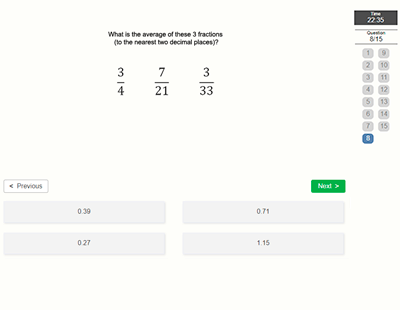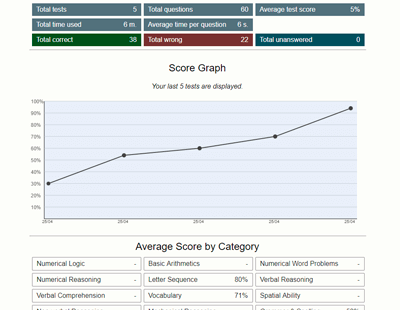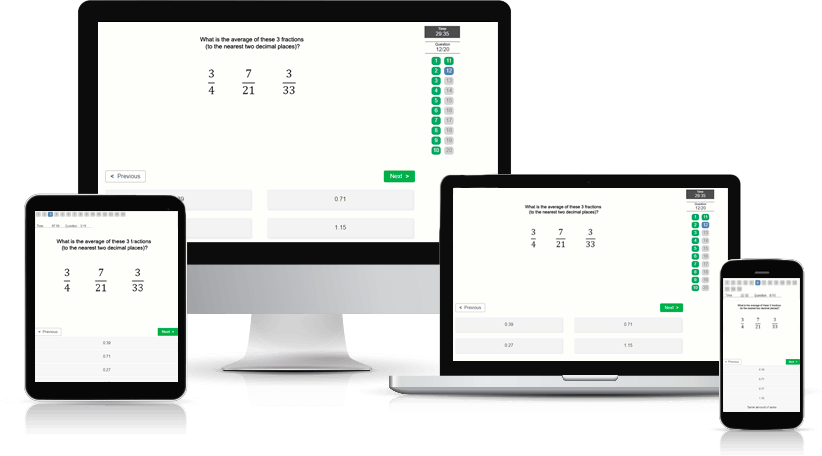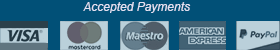﻿ Basic Arithmetic Test - Aptitude-Test.com

# Basic Arithmetic Test

A basic arithmetic test will challenge your skills and knowledge of the most basic mathematics. Due to the purpose of the test, calculators are never allowed for these tests, although using pen and paper is recommended.

What questions can I expect?
In a basic arithmetic test you are required to solve simple mathematical equations and answer questions regarding basic mathematics. When facing a basic arithmetic test, we suggest that you brush up on the following topics:

• Order of Operation
• Fractions
• Decimals
• Percentages
• Square roots
• Powers and exponents
• Conversions
• Measurement, including money, distance, area, and volume
In addition to these topics, we suggest that you practice your mental calculation of addition, subtraction, multiplication, and division. Being able to make fast and accurate calculations mentally will improve your performance significantly. With our Test Prep Account you will get access to our Math Knowledge Study Tool, which will help in your preparation, as well as our practice questions.

Where are Basic Arithmetic tests used?
These tests are frequently used as pre-employment assessments for occupations requiring basic mathematical skills. These occupations include: clerks, cashiers, receptionists, teachers, and more.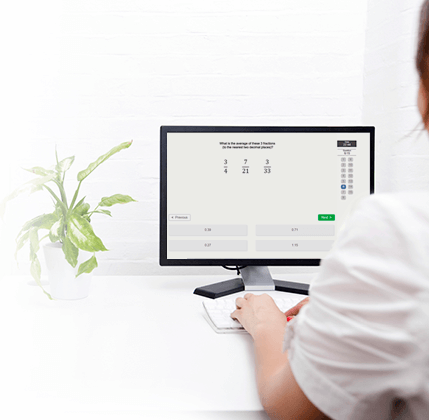## Practice Basic Arithmetic Test

Try a free Basic Arithmetic Test.
This test contains 6 test questions and has a time limit of 6 minutes.

##### Would you like to improve your test score? Practice smart with a Test Prep Account.

Practice on 110 Basic Arithmetic and more than 420 other numerical aptitude test questions with detailed description and score statistics.

The many features of a Test Prep Account:

• Preparation software developed under a didactic and methodical perspective
• High-quality practice test questions
• Clearly explained solutions
• Accessible on all devices
• Detailed score data and progression charts
• Reference scores to compare your performance against others
• Training Assistant
• Practice mode (feedback after every question and no time limit)